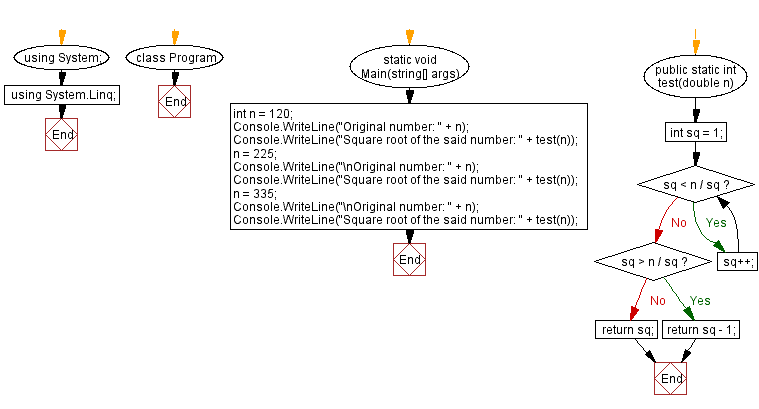﻿ C# - Square root of a given number# C# Sharp Exercises: Square root of a given number

## C# Sharp Basic: Exercise-93 with Solution

Write a C# Sharp program to calculate the square root of a given number. Do not use any built-in-function, return integer part of the result.

Sample Data:
(120) -> 10
(225) -> 15
(335) -> 18

Sample Solution:

C# Sharp Code:

``````using System;
using System.Linq;
namespace exercises
{
class Program
{
static void Main(string[] args)
{
int n = 120;
Console.WriteLine("Original number: " + n);
Console.WriteLine("Square root of the said number: " + test(n));
n = 225;
Console.WriteLine("\nOriginal number: " + n);
Console.WriteLine("Square root of the said number: " + test(n));
n = 335;
Console.WriteLine("\nOriginal number: " + n);
Console.WriteLine("Square root of the said number: " + test(n));
}
public static int test(double n)
{
int sq = 1;
while (sq < n / sq)
{
sq++;
}
if (sq > n / sq) return sq - 1;
return sq;
}
}
}
``````

Sample Output:

```Original number: 120
Square root of the said number: 10

Original number: 225
Square root of the said number: 15

Original number: 335
Square root of the said number: 18
```

Flowchart:C# Sharp Code Editor:

Improve this sample solution and post your code through Disqus

Previous C# Sharp Exercise: Next prime number of a given integer.
Next C# Sharp Exercise: Find the longest common prefix from an array of strings.

What is the difficulty level of this exercise?

﻿